## Programming Multi Agent Simulation

If you are not familiar with MS Excel, you may want to read this section to get the detail step by step instruction on how to build the multi agent simulation in Microsoft Excel from the scratch. The explanation on the principle of the program has been explained in the last section. Description on how to use the program can be found below:

First, create a cell in C1 and give name "Control". To give a name to cell or a block of a cell, we can simply type the name on the name box that usually contain coordinate.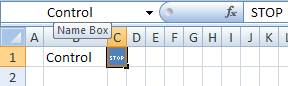Then, enable the iteration calculation from Tools > Options > Calculation tab in MS Excel 2003 or office button > Excel Options > Formulas Tab in MS Excel 2007. Check the enable the iteration calculation and set the maximum iteration as 1. Using MS Excel iteration will avoid Circular reference warning when your formula refers to it own cells.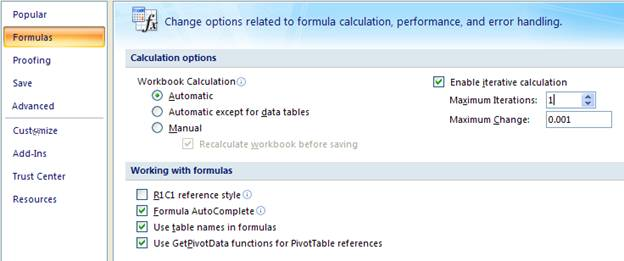Now you can create the simulation clock. Suppose we will create it in cell AF1. Type =IF(Control="",simClock+1,0) in cell AF1 and give name "simClock" to that cell. You can test if the formula work simply by deleting the content of cell control C1 and press F9 repeatedly. The simulation clock counter will start to increase its number every time you press F9.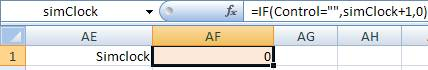Next we are going to create a single agent by setting the agent's properties. The first property represents agents' decision to move. Suppose we will create this property in cell AE5, thus type =IF(RAND()<0.5,1,0) in that cell AE5.

The second agent's property is the current cell position where the agent exists. In our simple model, there are three factors that influence the movement of agents: agent's decision, position of finishing line and whether the race has begun. The current cell position of the agent depends on the agent's mind on its decision to move. If the agent decide to move (that is cell AE5 contains 1), then the agent position must be advanced. Another criterion for the movement is the finishing line (in this case, suppose we use position number 25 as the last cell). If agent's position is still below 25, then the agent still can move. The race begins when the content of the Control cell is deleted by user. Suppose the current cell property will be stored in cell AF5, then type =IF(Control="",IF(AND(AE5=1,AF5<25),AF5+1,AF5),0) in cell AF5.

The last agent's property is useful to record the time of the race. Suppose the last cell of the race field is cell AB5 and we will store the racing clock property of the agent in cell AG5. In cell AG5, type =IF(AB5=0,simClock,AG5) . The formula says that if the agent has not reach the finish line of the race field, copy the current simulation clock, otherwise copy the last simulation clock when the agent reach the finishing line.

After that, you can copy row AE5 to AG5 to row AE6 to AG9. Each row represent one agent, thus now you have 5 agents. The formulas are shown below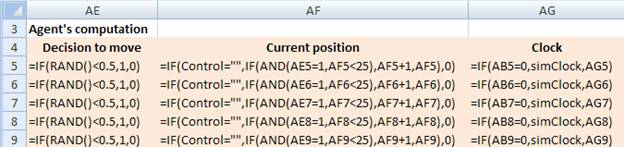By now you are ready to create the race field for the animation of the race. First, put the position number in C4 as zero, then in D4 you type =C4+1 and copy cell D4 into cell E4 to AB4.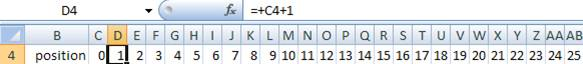Then, type in cell C5: =IF(\$AF\$5=C\$4,1,0) . In this case, cell AF5 represent the current position the agent. Two \$ signs make the coordinate fixed when you copy to the right and to rows down. C4 is the position number of the race field. Single \$ sign on the number is used to fixed the row when you copy the formula. Then, you copy the formula from cell C5 into C5 to AB9.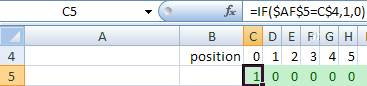To make the animation nicer, you can set conditional formatting. For example, using icon sets in MS Excel 2007 or using Fill cell color in MS Excel 2003.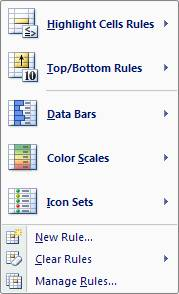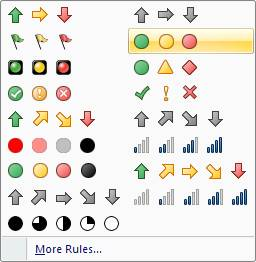The final race field become: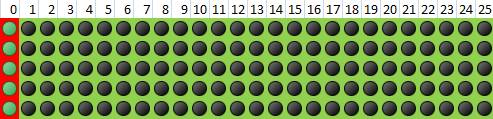The last thing you may want to do is to obtain the winner of the race. In this case, we simply compute the rank of the racing clock property of each agent. Type in cell AC5: =RANK(AG5,AG\$5:AG\$9,1) , and then copy from cell AC5 to cell AC6 to AC9.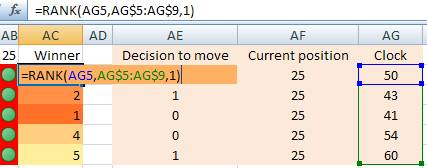In this tutorial, you have learned a basic multi agent model using mere spreadsheet (without any programming). Of course, the agents behave only single directional movement and it would be your challenge to improve the agent behavior into more intelligence. Compare to code it into other a programming language (such as C++ or Java), playing around in MS Excel is much easier and faster to develop.

Acknowledgement :

Thanks to Jon Fernandez to raise the challenge on how to create a race of multi agent using MS Excel.